Question

# Probability

A multiple-choice test has 30 questions and each one has five possible answers, of which one is correct. If all answers were guesses, find the probability of gettingexactly four correct answers. (Points : 3)
.0604
.1325
.2552
.8000

We need at least 10 more requests to produce the answer.

0 / 10 have requested this problem solution

The more requests, the faster the answer.

All students who have requested the answer will be notified once they are available.

#### Earn Coins

Coins can be redeemed for fabulous gifts.

Similar Homework Help Questions
• ### statistics

A multiple-choice test has 30 questions and each one has five possible answers, of which one is correct. If all answers were guesses, find the probability of gettingexactly four correct answers. (Points : 3).0604.1325.2552.8000

• ### 10. A multiple-choice test has 30 questions and each one has five possible answers, of which one...

10. A multiple-choice test has 30 questions and each one has five possible answers, of which one is correct. If all answers were guesses, find the probability ofgetting exactly four correct answers. (Points : 3).0604.1325.2552.8000

• ### QUESTION 14 QUESTION 14 Find the indicated binomial probability. Round to five decimal places when necessary....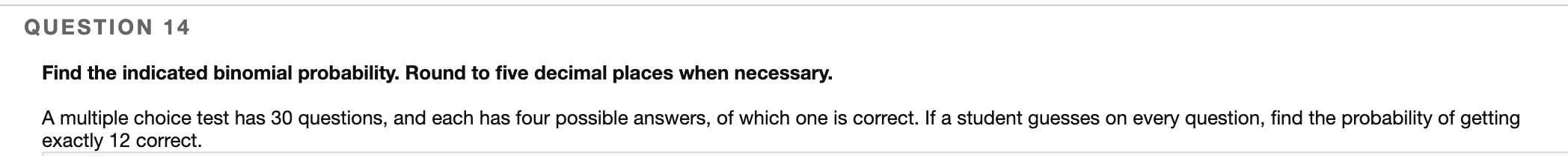QUESTION 14 QUESTION 14 Find the indicated binomial probability. Round to five decimal places when necessary. A multiple choice test has 30 questions, and each has four possible answers, of which one is correct. If a student guesses on every question, find the probability of getting exactly 12 correct.

• ### Find the indicated probability, A multiple choice test has 8 questions each of which has 4...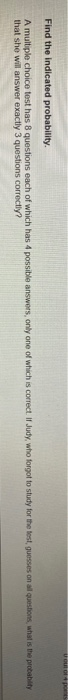Find the indicated probability, A multiple choice test has 8 questions each of which has 4 possible answers, only one of which is correct. Il Judy, who forgot to study for the test guesses on all questions, what is the probability that she will answer exactly 3 questions correctly?

• ### indicated probability by using the normal distribution as an approximation to the binomial distribution. 12) A...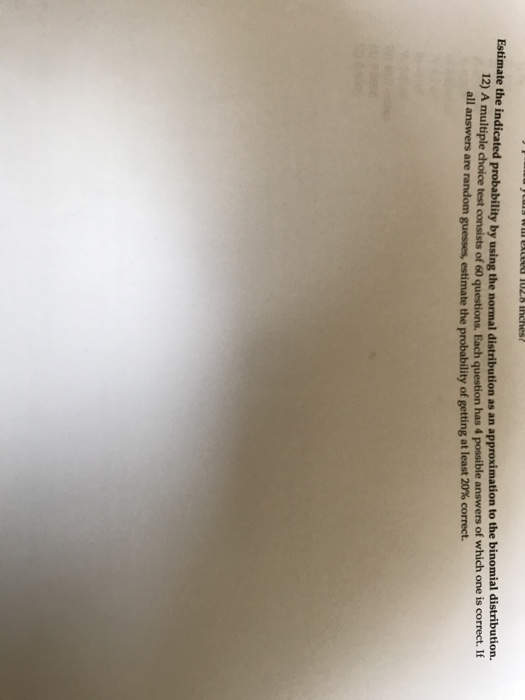indicated probability by using the normal distribution as an approximation to the binomial distribution. 12) A multiple choice test consists of 60 questions. Each question has 4 possible answers of which one is correct. If Estimate the all answers are random guesses, estimate the probability of getting at least 20% correct.

• ### find the indicated probability

A multiple choice test has 10 questions each of which has 4 possible answers, only one of which is correct. If Judy, who forgot to study for the test, guesses on allquestions, what is the probability that she will answer exactly 3 questions correctly?a-0.2816b-0.0021c-0.5006d-0.0156e-0.2503

• ### Estimate the indicated probability by using the normal distribution as an approximation to the binomial distribution...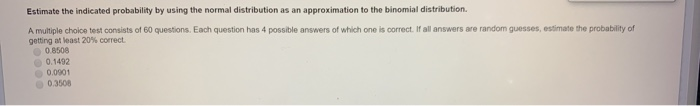Estimate the indicated probability by using the normal distribution as an approximation to the binomial distribution A multiple choice test consists of 60 questions. Each question has 4 possible answers of which one is correct. If all answers are random guesses, estimate the probability of getting a least 20% correct 0.8508 0.1492 0.0001 03505

• ### A multiple choice test has 10 questions each of which has 4 possible answers, only one...

A multiple choice test has 10 questions each of which has 4 possible answers, only one of which is correct. If Judy, who forgot to study for the test, guesses on all questions, what is the probability that she will answer exactly 3 questions correctly?

• ### I'm confused by how to start this probability problem 43. A ten question multiple-choice test with...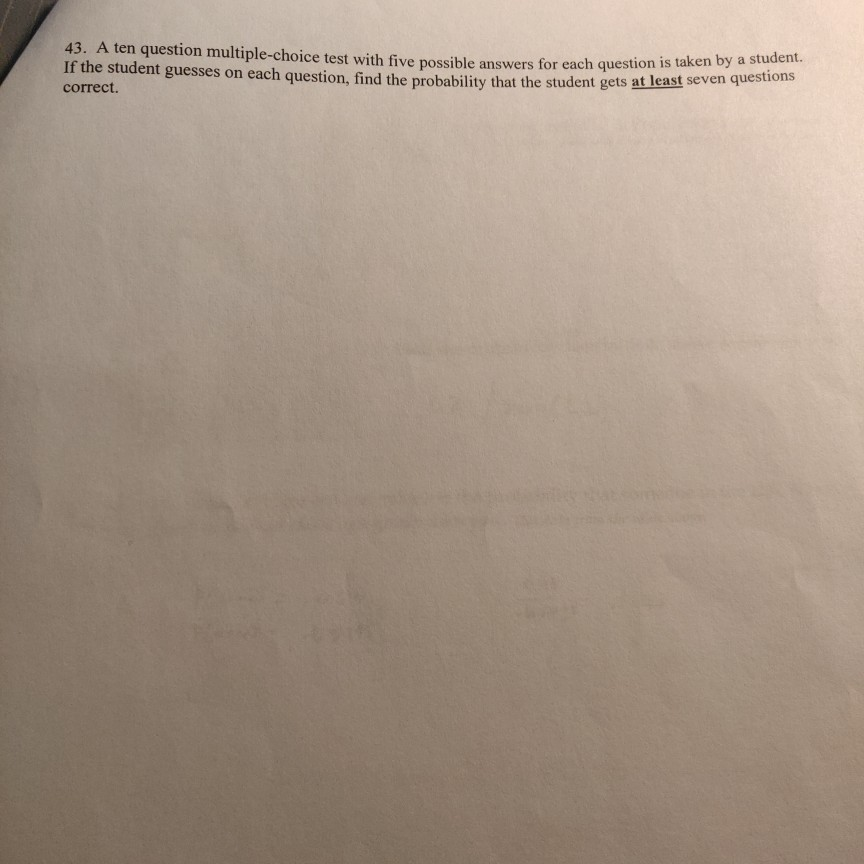I'm confused by how to start this probability problem 43. A ten question multiple-choice test with five possible answers for each questo If the student guesses on each question, find the probability that the stud ossible answers for each question is taken by a student. ach question, find the probability that the student gets at least seven questions correct.

• ### Find the indicated probability. Find the probability of correctly answering the first 3 questions on a...Find the indicated probability. Find the probability of correctly answering the first 3 questions on a multiple choice test if random guesses are made and each question has 6 possible answers, 2 216